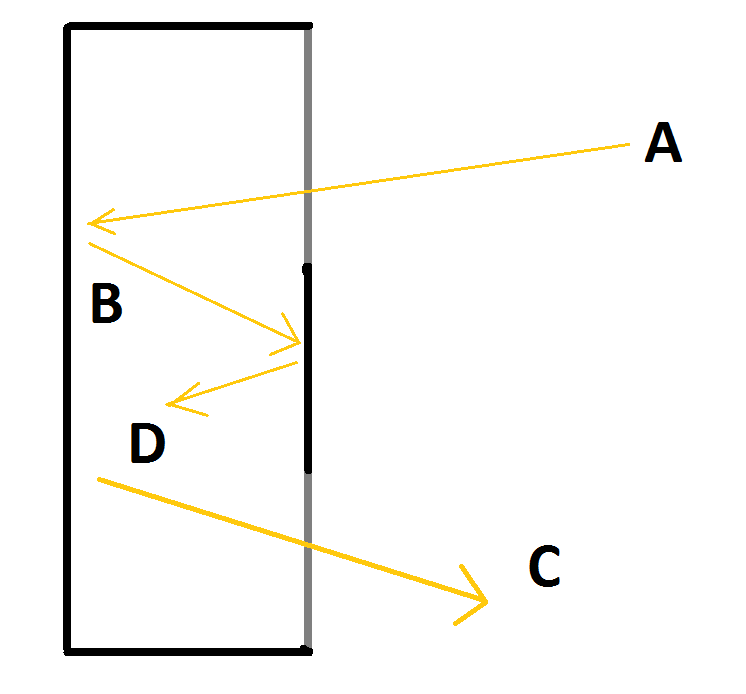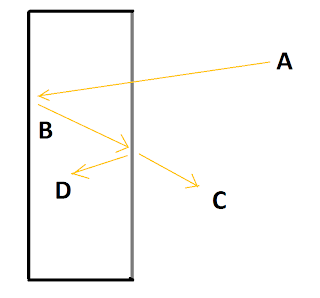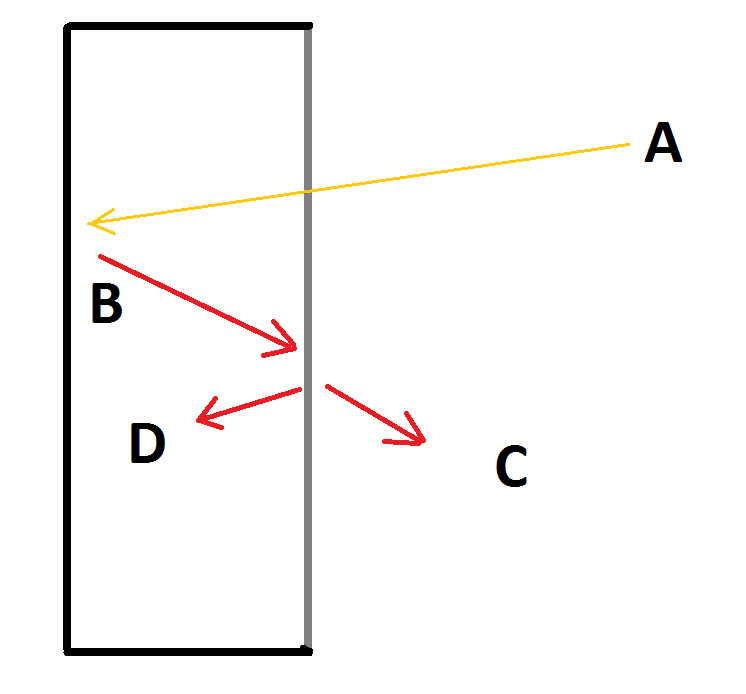## Saturday, March 19, 2011

### The Greenhouse Effect and the Laws of Thermodynamics

I have just made a comment at Skeptical Science which I believe provides a good summary refutation of the denier claim that the greenhouse effect violates the laws of thermodynamics. Here it is:

For anybody not convinced of AGW who has been following this discussion, consider the following model:In this model we have light entering a box (A) at an angle. It then strikes a mirror on the back of the box, is reflected of a mirror on the front of the box so placed that it does not block any incoming light. The now twice reflected light returns to the back of the box, striking the mirror once more. It then goes through a second transparent zone in the front of the box.

Assume the ideal case in which all apertures are 100% transparent, all mirrors are 100% reflective and flat, and in which the light is so angled that neither the incoming beam (A) nor the outgoing beam (C) strike any part of the mirror on the top of the box. In that case, after sufficient time for light to transit the box three times, and with a constant light source providing beam (A), then the box will have the following equalities:

1) Incoming light (A) = Outgoing light (C) (by virtue of conservation of energy).

2) Light reflected from the lid (D) = Outgoing light (C).

3) Light reflected from the back = light striking the underside of the lid (B) = light transmitted by the lid (C) plus light reflected by the lid (D) (by virtue of conservation of energy). This may require a little clarification. The lid consists of three sections in this model, two transparent, and one mirrored on the underside. One transparent section allows in the incoming light (A). The other allows out the outgoing light (C). So the light striking the back of the lid equals the light striking the mirrored section plus the light striking the transparent section through which the light exits.

Therefore
4) Light reflected from the back of the box (B) = light reflected from the back of the lid (D) plus Incoming light (A) = 2 x A (again, by conservation of energy.

Such a box clearly does not violate any law of thermodynamics. If it did violate such laws, so much the worse for those laws, for the box could be set up as a primary school science project, and will behave as advertised. As I assume that the scientists of the world are not part of a massive conspiracy to foist upon us "laws" that can be refuted by any primary school kid with a plate of glass and two mirrors, I will take it that this box does not violate the laws fo thermodynamics is beyond dispute.

However, having said that, we should notice that in each time interval equal to the time it takes a photon to travel from the lid to the back wall:

5) the accumulated photons at equilibrium is 4 times the number of photons that enter the light box at each time interval; and

6) at equilibrium the number of photons reflected in each time interval is 3 times the number that enter the light box in each time interval. Of those, 2 times that number are reflected of the back wall, and a number of photons equal to the number that enter are reflected of the lid. This ignores reflections of the side walls which are irrelevant to the overall issue.

Now, reconsider this model:

The description of the model is found in comment 615 above. Essentially, however, it is the same model as above, except the lid is replaced by a lid which lets in all light from outside and reflects exactly half of light from the inside, transmitting the remainder. The walls are perfectly mirrored.After equilibrium is established (ie, in approximately 20 times the time it takes for light to travel from the lid to the back of the box, see Phil's comment @624 and my comments @626 and@640 above), the equalities (1) to (4) above hold of this box as well (with appropriate adjustment of wording - for exact wording see comment 615 above).

However, the facts (5) and (6) also hold of this box (see comment 647 above). Now, these two facts, according to some people, create a problem for this model. According to those people, they cause it to violate the laws of thermodynamics. But as we have seen, our first model (in this comment) has exactly these two properties, and does not violate the laws of thermodynamics. Therefore this model does not violate the laws of thermodynamics either. In fact, the only interesting difference between this model and the previous one is that this model takes about five times as long to reach equilibrium.

So, having established beyond reasonable doubt that the simple light box does not violate the laws of thermodynamics, consider the following model:This model is quite different from the preceding two. For a start, (in the ideal case) the back and walls are perfectly absorbing on the inside, and perfectly reflective at all wavelengths on the outside. This means any light entering the box will be absorbed by the box, warming the back of the box. We also suspend the box in a vacuum so that energy can only escape by radiation. Because the outside of the walls and back are perfectly reflective, this means they have zero emissivity and energy can only escape through the lid.

The lid itself has (in the ideal case)perfect thermal isolation from the sides, and back (which means the box contains a vacuum). It is perfectly transparent to all light below a certain wavelength, and perfectly absorbing for all wavelengths above that amount. It (and the back and walls of the box) is also a perfect thermal conductor so that its outside and inside have exactly the same temperature.

Importantly, the wavelength of the incoming light is less than the critical wavelength of the lid. Also, for simplicity, the total energy of the incoming light is less than the amount at which the shortest wavelength of a black body emission from the back and sides of the box is shorter than the critical wavelength.

Given these conditions, when you start shining light on the box, the back and sides will heat up and start emitting long wave length radiation. Because all of that radiation has a wavelength longer than the critical value of the lid, the lid will in turn start heating up and emit radiation. Eventually the energy emitted outwards (C) will equal the energy of the incoming light (A), at which time equilibrium has been reached.

At that time, the following equalities will hold:

1) Incoming energy (A) = Outgoing energy (C) (by virtue of conservation of energy).

2) energy radiated from the lid inwards(D) = Outgoing energy (C) (by virtue of both sides of the lid having the same temperature and emissivity).

3) energy radiated from the back = energy absorbed by the underside of the lid (B) = energy radiated outwards by the lid (C) plus energy radiate inwards by the lid (D) (by virtue of conservation of energy).

Therefore
4) Energy radiated from the back of the box (B) = energy radiated inwards by the lid (D) plus Incoming energy (A) = 2 x A (again, by conservation of energy).

It will also be true that:

5) The accumulated energy in the box in the form of radiation will be four times the energy carried into the box by incoming light in the time it takes for light to travel from the lid to the back of the box.

Indeed, an additional amount of energy will be stored in the back, sides and lids of the box depending on their heat capacity. That means the greater their heat capacity, the longer the box will take to reach equilibrium. That is simple a consequence of the incoming energy being diverted to heat the wall and lid, and therefore not being available to leave the box. It is only when the walls and lid reach a stable temperature that the energy is no longer used in the box, and will leave at the same rate it entered.

Now, very clearly, these 5 facts mirror the first 5 facts in our first, and second model. Therefore, if those five facts do not cause the first two models to violate the laws of thermodynamics (which they do not), then these facts cannot cause the third model to violate the laws of thermodynamics. In fact, the only thermodynamically interesting difference between the first two and the third model is that in the first two, the leaving energy had an almost identical entropy to the incoming energy, whereas in this model, the leaving energy has a much higher entropy in virtue of its much longer wavelengths.

The other interesting fact about this model is that it is an example of (not an analogy to, but an example of) the green house effect. It is a very simple example, but example it is none-the-less. And as this example does not violate any law of thermodynamics, it follows that the greenhouse effect does not violate any law of thermodynamics.

QED